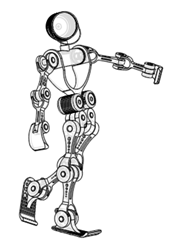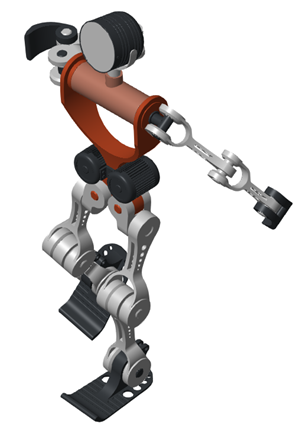## Import a URDF Humanoid Model

### URDF Import

You can import a URDF model into the Simscape™ Multibody™ environment. The import process occurs in a single step based on the `smimport` function. The `smimport` function converts the URDF model directly into an equivalent Simscape Multibody model.

### Example Overview

This example shows how to import an Onshape® model of a humanoid robot assembly. The model comprises various parts (”links” in URDF jargon) representing the torso, head, and limbs of the robot. The parts connect through revolute and weld joints (”continuous” and “fixed” respectively). This model is identical to that shown in Import an Onshape Humanoid Model. You can open the model from the MATLAB® command prompt by entering the command:

`open sm_humanoid.urdf`

Model Schematic### Import the Model

Use the `smimport` function to import the URDF model:

```urdfModel = 'sm_humanoid.urdf'; smimport(urdfModel);```

The function generates a Simscape Multibody model of the humanoid robot. The file extension is required to identify the import file as URDF. Update the imported model (in the Modeling tab, click .) to open a static visualization in the initial state. The figure shows the results.Build on the model, for example, by adding control systems to actuate the various joints. For a controlled example, at the MATLAB command prompt enter `sm_import_humanoid_urdf`. Simulate the model to view a simple animation.